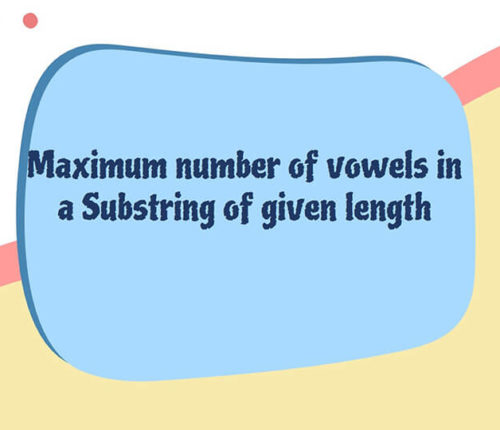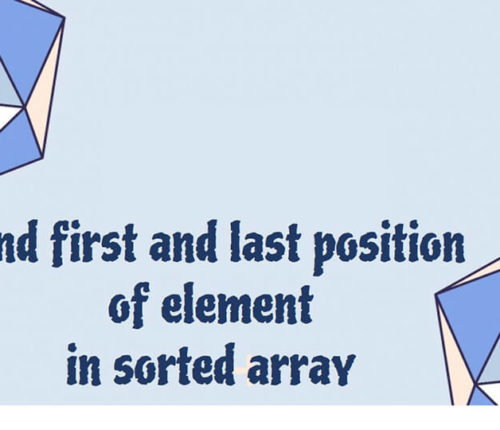# Sort an array of 0s, 1s and 2s

If you want to practice data structure and algorithm programs, you can go through Java coding interview questions.

In this post, we will see how to sort an array of 0s, 1s and 2s.We have already seen a post on sort 0s and 1s in an array.

## Problem

Given an array containing zeroes, ones and twos only. Write a function to sort the given array in `O(n)` time complexity.

Input :
[1, 2, 2, 0, 0, 1, 2, 2, 1]

Output :
[0, 0, 1, 1, 1, 2, 2, 2, 2]

## Solution

APPROACH – I :
A very basic approach to solve this problem can be keeping the count of number of zeroes, ones and twos in the given array and then manipulate the given array in accordance with the frequency of every number. This approach is a bit inspired by counting sort. No matter what the initial value of that particular index is, we first put all the zeroes we have in the array starting from index zero, then put all the ones and after that put all the twos.

Steps:
1.) Traverse the given array once and keep incrementing the count of the number encountered.
2.) Now Traverse the array again starting from index zero and keep changing the value of the element on current index first exhaust all the zeroes then ones and finally all the twos.

This way we have a sorted array where all the zeroes are in starting followed by all the ones and then in last section we have all the twos in a time complexity of `O(n)`.

• But the major drawback of this approach is, we have to traverse the given array twice once for counting the number of zeroes, ones and twos and second one for manipulating the array to make it sorted, which can be done only in a single pass.
APPROACH – II :
This algorithm is called as `Dutch national flag algorithm `or` Three way partitioning` in which elements of similar type are grouped together and their collective groups are also sorted in a the correct order.
Now we have three types of elements to be sorted, therefore, we divide the given array in four sections out of which 3 sections are designated to `zeroes`, `Ones` and `twos` respectively and one section is `unknown` or the section which is left to be explored. Now for traversing in these sections we need 3 pointers as well which will virtually divide the given array in four segments.
Let us name these pointers as low, mid and high.

Now we can tell the starting and ending points of these segments.

• Segment-1 : zeroes
This will be a known section containing only `zeroes` with a range of `[0, low-1]`.
• Segment-2: Ones
This will also be a know section containing only ones with a range of `[low, mid-1]`.
• Segment-3 : Unexplored
This will be an unknown section as the elements in this sections are yet to be explored and hence it can contain all types of element that is, zeroes, ones and twos. Range of this segment will be `[mid, high]`
• Segment-4 : Twos
This will be the last and known area containing only twos having the range of `[high+1, N]` where N is the length of the given array or basically the last valid index of the given array.

Steps used in this Algorithm to sort the given array in a single pass :

(i) Initialize the low, mid and high pointers to, `low = 0`, `mid = 0`, `high = N`
(ii) Now, run a loop and do the following until the `mid` pointer finally meets `high` pointer.As the `mid` pointer moves forward we keep putting the element at `mid` pointer to its right position by swapping that element with the element at pointers of respective sections.
(iii) CASE – I : If the element at `mid`, that is, `A[mid] == 0`, this means the correct position of this element is in the range `[0, low-1]`, therefore, we swap `A[mid]` with `A[low] `and increment low making sure that element with index lesser than low is a Zero.
(iv) CASE – II : If the element at `mid`, that is, `A[mid] == 2`, this means the correct position of this element is in the range `[hi+1, N]`, therefore, we swap `A[mid]` with `A[hi]` and decrement high making sure that element with index greater than high is a two.
(v) CASE – III : If the element at mid, that is, `A[mid]=1`, this means that the element is already in its correct segment because `[low, mid-1]` is the range where it needs to be. Therefore, we do nothing and simply increment the mid pointer.

So, there are total three cases, let us take a moment and emphasise on the fact that mid pointer gets only incremented only when the element `A[mid] == 1`.
Let us discuss every case individually,

For case – I : In this case we increment `mid` as well along with increment `low` pointer, as we are sure that element at low pointer before swapping can surely only be one as had it been a two, it would have already got swapped with `high` pointer when `mid` pointer explored it as the only reason that mid pointer left it because it was a one.

For case – II : Now, In this case we swap the element at `mid` and `high`, but unlike case – I, in this case we are not sure about the element which will come at `mid` index after swapping as the element at `high` index before swapping can be any of zero, one or two, therefore, we need to explore this swapped element and hence we do not increment `mid` pointer in this case.

For case – III : There is no confusion regarding incrementing `mid` in this case as already discussed, as we know the element at `mid` is one therefore we definitely need to increment mid here.

Time complexity of this algorithm is also O(n) but it sorts the array in just a single pass and without any extra space unlike previous approach.

That’s about sort an array of 0s, 1s and 2s.

import_contacts

### You may also like:## Related Posts

•18 June

### Maximum Number of Vowels in a Substring of Given Length

Table of ContentsApproach – 1 Generate All Substrings Using substring() MethodApproach – 2 Using Sliding Window Method (Linear Time Solution) In this article, we will look at an interesting problem related to the Strings and [Sliding-Window Algorithm](https://java2blog.com/sliding-window-maximum-java/ “Sliding-Window Algorithm”). The problem is : "Given a String we have to Find the Maximum Number of Vowel […]

•04 June

### Search for a range Leetcode – Find first and last position of element in sorted array

Table of ContentsApproach 1 (Using Linear Search)Approach 2 (Using Modified Binary Search-Optimal) In this article, we will look into an interesting problem asked in Coding Interviews related to Searching Algorithms. The problem is: Given a Sorted Array, we need to find the first and last position of an element in Sorted array. This problem is […]

•30 April

### Convert Postfix to Infix in Java

Learn about how to convert Postfix to Infix in java.

•30 April

### Convert Prefix to Postfix in Java

Learn about how to convert Prefix to Postfix in java.

•16 April

### Data Structures in java

•